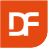Can't find what you are looking for? Try these pages!

Products

# Free DataFlex Conversions Library for DataFlex 2022August 31, 2022

With the DataFlex Conversions Library you can convert from one unit to another. For example Fahrenheit to Celsius. This free library is now available for DataFlex 2022!

## The DataFlex Conversions Library

Many developers need to have functions to convert one unit to another. The DataFlex Conversions Library contains a number of classes with the functions to convert. Available conversions are:

• Viscosity - Converts for example kg/m·s to N·s/m2
• Angle - Converts for example degrees to minutes of arc
• Array - Converts for example the Equal rank to Element Data Type
• Bit - Converts for example bits to bytes or kilobytes
• Energy - Converts for example BTU to joules
• Hex - Converts for example Hex to decimal
• Kinematic Viscosity - Converts for example m²/sec to m²/min
• Length - Converts for example centimeters to meters
• Oct - Converts for excample octal to decimal
• Power - Converts for example BTU per second to horse power
• Pressure - Converts for example pascal to bar
• Temperature - Converts for example Fahrenheit to Celsius
• Time - Converts for example minutes to hours
• Velocity - Converts for example meters per second to feet per second
• Volume - Converts for example liter to milliliter
• Weight - Converts for example kilogram to gram

## Use the DataFlex Conversions Library